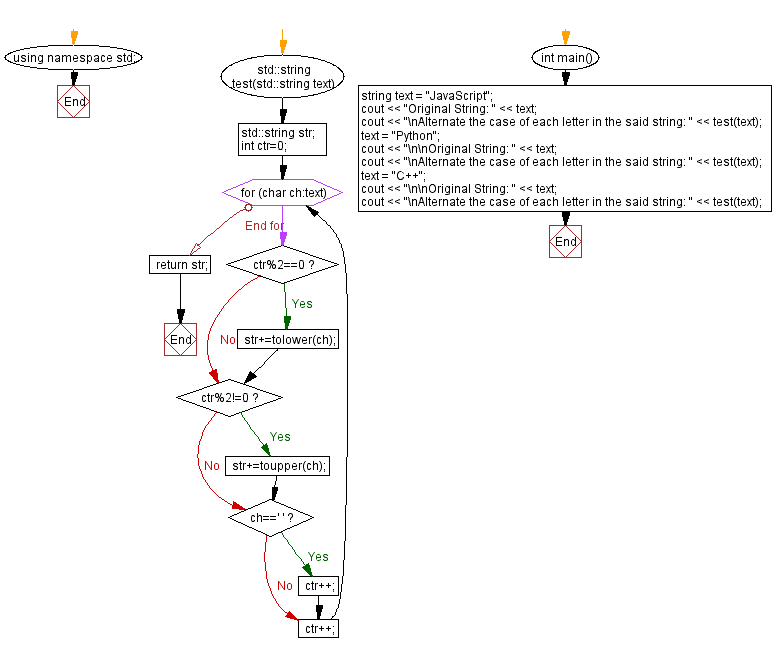﻿ C++ - Alternate the case of each letter in a string

# C++ String Exercises: Alternate the case of each letter in a string

## C++ String: Exercise-42 with Solution

Write a C++ program that alternates the case of each letter in a given string of letters.
Pattern: First lowercase letter then uppercase letter and so on.

Test Data:
("JavaScript") -> "jAvAsCrIpT"
("Python") -> "pYtHoN"
("C++") -> "c++"

Sample Solution:

C++ Code:

``````#include <bits/stdc++.h>

using namespace std;
std::string test(std::string text) {
std::string str;
int ctr=0;
for(char ch:text){
if(ctr%2==0)
str+=tolower(ch);
if(ctr%2!=0)
str+=toupper(ch);
if(ch==' ')
ctr++;
ctr++;
}
return str;
}int main()
{
string text = "JavaScript";
cout << "Original String: " << text;
cout << "\nAlternate the case of each letter in the said string: " << test(text);
text = "Python";
cout << "\n\nOriginal String: " << text;
cout << "\nAlternate the case of each letter in the said string: " << test(text);
text = "C++";
cout << "\n\nOriginal String: " << text;
cout << "\nAlternate the case of each letter in the said string: " << test(text);
}
``````

Sample Output:

```Original String: JavaScript
Alternate the case of each letter in the said string: jAvAsCrIpT

Original String: Python
Alternate the case of each letter in the said string: pYtHoN

Original String: C++
Alternate the case of each letter in the said string: c++
```

Flowchart:C++ Code Editor: